Latest Banking jobs   »   IBPS RRB 2021 Notification Out PDF   »   Quantitative Aptitude Quiz For RRB PO...

# Quantitative Aptitude Quiz For RRB PO Mains 2021- 8th September

Directions (1 – 5): Table given below shows data of five institutes regarding number of girls students, percentage of boys and number of students (boys + girls) under one mentor. Study the data given below carefully and answer the following questions.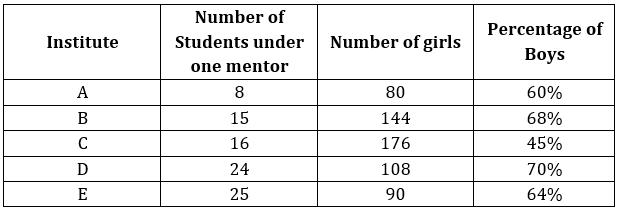Q1. Number of mentors in institute D is what percent more/less then number of mentors in institute A?

(a) 20%

(b) 160%

(c) 80%

(d) 60%

(e) 40%

Q2. If in institute E, girls students increases by 60% and boys students increases by 35% while number of students under one mentor increases by 20% then how many more mentors are required in institute E?

(a) No more mentors required

(b) 1

(c) 2

(d) 3

(e) 4

Q3. Total number of students in institute B and C together is how much more than total number of students in institute D and E together?

(a) 130

(b) 140

(c) 150

(d) 160

(e) 170

Q4.Out of total mentors in institute C, 65% are females, then find the number of male mentors in institute C.

(a) 5

(b) 7

(c) 9

(d) 11

(e) 13

Q5. Total number of students in another institute F is 15% more than that of in institute D, while number of students under one mentor is 20% more than that of in institute B. Find number of mentors required in institute F.

(a) 17

(b) 19

(c) 21

(d) 23

(e) 25

Directions (6-10): Pie-chart given below shows population of two cities travel by five modes of transportation. Study the chart carefully and answer the following question.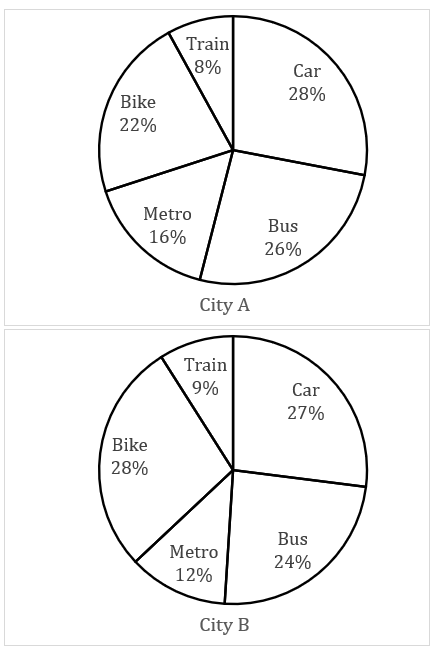Note:- Ratio of total population of city A to city B is 5 : 2.

Q6. Total number of person travel by bike in city A is 945 more than that of in city B, then find the total number of person who travel by bus in city A and city B together?

(a) 3010

(b) 3115

(c) 3055

(d) 3085

(e) 3145

Q7. Number of females travel by car in city B is 25% more than number of males travel by car in city B, which is 25% of number of males travel by car in city A. If total number of person travel by metro in city B is 456, then find the number of females travel by car in city A.

(a) 570

(b) 1140

(c) 1824

(d) 836

(e) 912

Q8. If total number of person travel by car from city A and city B together is 582, then total number of person travel by bike and metro together from city A is what percent more than total number of person travel by bike and metro together from city B?

(a) 37.5%

(b) 50%

(c) 137.5%

(d) 150%

(e) 237.5%

Q9. If number of person travel by car in city B is 126 more than number of person travel by train in city A, then find the average number of person travel by metro in city A and B together?

(a) 468

(b) 364

(c) 414

(d) 428

(e) 442

Q10. Total number of person in city B who travel by metro and train together is how much more than total number of person in city A who travel by train. If it is given that total population of city B is 95 more than total number of person in city A who travel by bike and metro together.

(a) 13

(b) 15

(c) 17

(d) 19

(e) 21

Solutions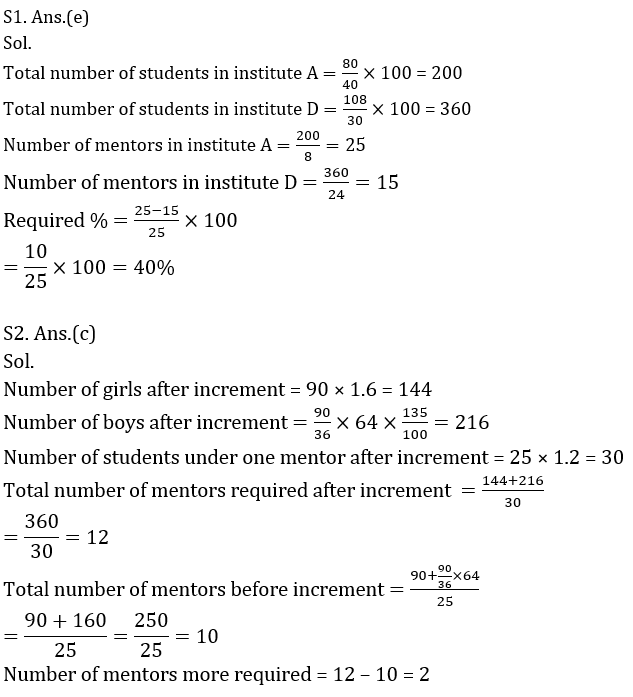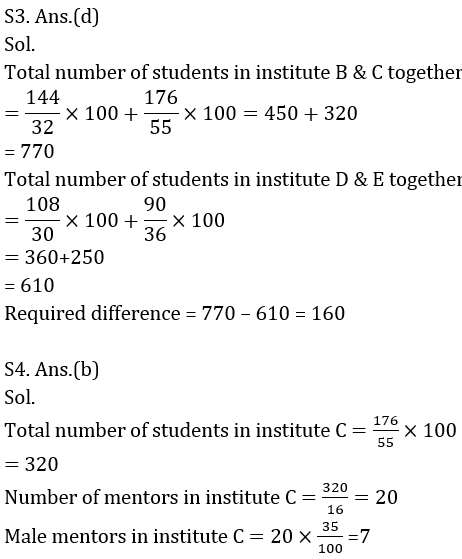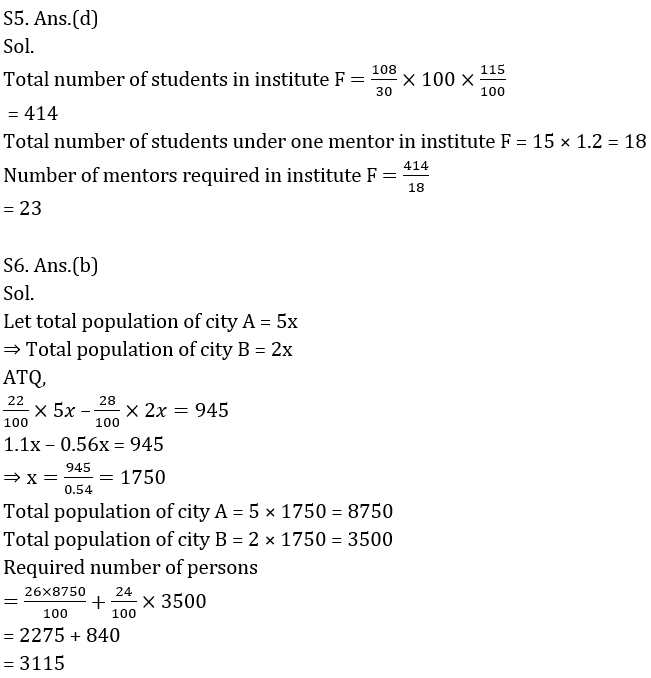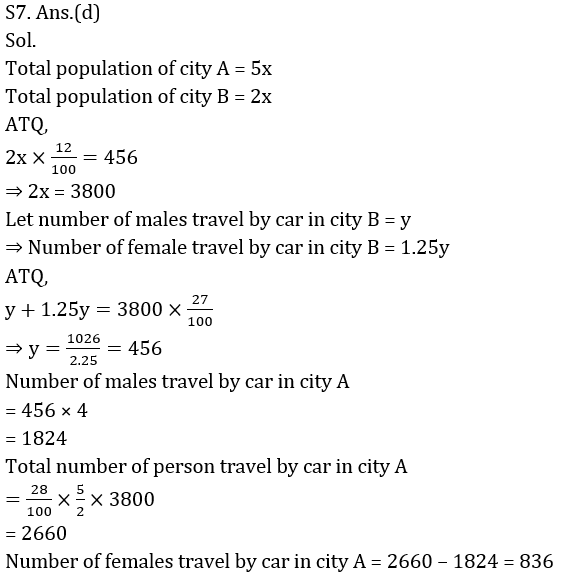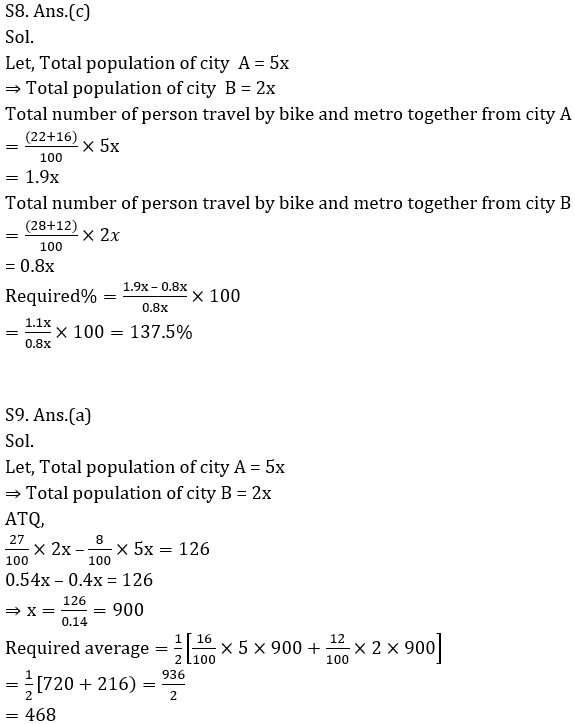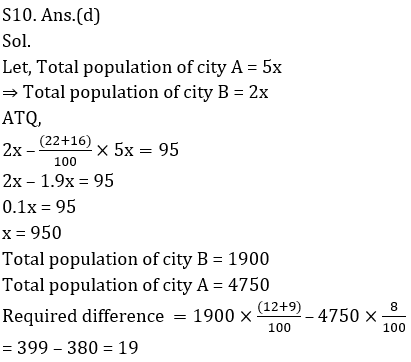#### Congratulations!Union Budget 2023-24: Free PDF